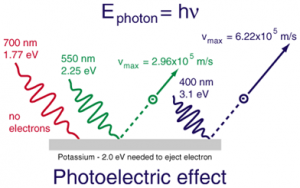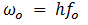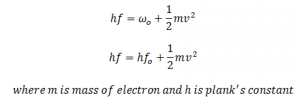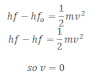# Einstein’s Photoelectric EquationWhen light of suitable frequency incident on the metal surface, a part of its energy is used for ejecting the electron just from the metal surface and remaining energy is used to move the emitted electron with certain velocity.
In fig, f is the frequency of incident light. So, its energy (hf) is used for two purposes; one is to eject the electron from metal surface which is equal to:And remaining is used to move the emitted electron which certain velocity v,
Mathematically,This equation is known as Einstein’s photoelectric equation.

From the above equation following conclusions can be drawn out:

• If the frequency of the incident ray of light is less than vo (fo) no photoelectric effect take place.
• IF f = f0, incident light just emits the electron from photo metal but velocity of emitted electron is zero.• If f > f0 photoelctric effect takes place and emitted electron moves with certain velcocity.
• Velocity of the emitted electron depends on the frequency of incident light but not on the intensity of light .
• The no. of emitted electrons depends on the intensity of light but not on the frequency of light.

Laws of photoelectric current:

• The rate of emission of electron from the metal surface is directly proportional to the intensity of light .
• The velocity of emitted electron depends on the frequency of the incident light .
• Photoelectric effect takes place only if the frequency of the incident light is greater than the threshold frequency. IT means energy of the incident light must be greater than the threshold energy of metal .
• Photoelectric effect is the instantaneous process which doesn’t depend on the temperature of the anode.